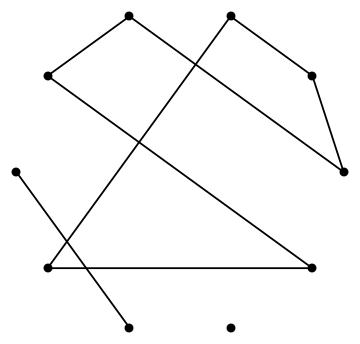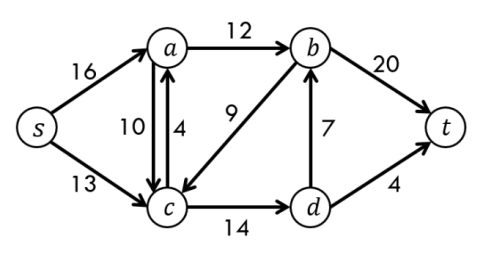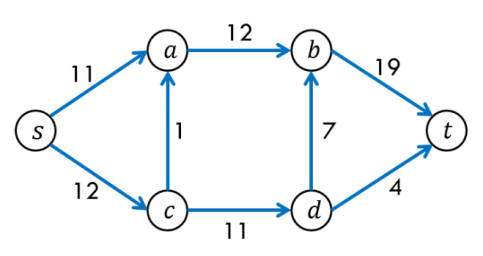Enrol now for our new online tutoring program. Learn from the best tutors. Get amazing results. Learn more.

June 26, 2022, 03:20:48 pm

###AuthorTopic: A quick glossary of graph/network concepts  (Read 4075 times)

0 Members and 1 Guest are viewing this topic.

#### RuiAce

• ATAR Notes Lecturer
• Moderator
• Great Wonder of ATAR Notes
••• Posts: 8814
• "All models are wrong, but some are useful."
• Respect: +2574##### A quick glossary of graph/network concepts
« on: June 07, 2019, 03:55:33 pm »
+15

Terminology can easily get the better of you in the graph theory/networks topics. The concepts may be solid to grasp, but I dare say it's easy to forget it all! So it may be easier to have all of those compiled together to help revise over them.

Please don't become reliant on this because you won't have it in the exam... it's your task to get used to seeing them all the time!

Basics
- The graph/network is the actual structure you're studying! In this course, you should be safe treating them synonymously.
- A vertex (sometimes called a node) is a point on the graph. Usually it is labelled.
- An edge on a graph joins two of its vertices together.

- A loop is an edge that joins a vertex back to itself. It's essentially an edge whose endpoints are the same vertex!

- The degree of a vertex is the number of edges incident on the vertex (i.e. end on the vertex). Always remember that loops add 2 to the degree!
-  One way I think about the degree is that it counts the number of ways you can "leave" a certain vertex. I always imagine that when we have a loop, we can leave the vertex by going along one end of the loop, and then the other. That way I never forget to address loops separately! (You don't have to do this if you hate this analogy though of course)

- If you already have a graph, a subgraph is nothing more than a (usually smaller) graph that's contained within it! You can get a subgraph by removing vertices, or edges, or both from your graph.

Some special graphs
- A simple graph is characterised by the absence of:
- Loops
- Any situation where two vertices are connected by more than one edge- Given $n$ vertices, the complete graph on $n$ vertices is a special simple graph, in which each vertex is connected to every other vertex by an edge. (Of course, because it is a simple graph, there will only be one edge joining each pair of vertices!)- A bipartite graph is hard to define (feels bad man). Essentially, the vertices of the graph can be split into two groups. The key property is that vertices in the same group can never be joined among each other by an edge. They can only be joined to vertices in the other group.- A directed graph is one in which each edge has a direction, usually denoted by an arrow on the edge. The assumption is that we can only go from a vertex $A$ to another vertex $B$, if an edge from $A$ to $B$ exists.

- A weighted graph is one in which each edge is assigned a number to (i.e. its weight).

Planar graphs
- A planar graph is one in which if we somehow rearrange the vertices and edges of the graph, we can get it so that no edges will cross over each other. (Sometimes we don't need to rearrange them - the edges may already not overlap.)- When drawn so that there are no overlapping edges, a face of a planar graph is essentially one of the regions cut by the edges in the graph. Always remember that there's always at least one face - the 'outside' region is also a face of the graph!

- Euler's formula states that $v + f - e = 2$ (but it is given on the formula sheet - yay!)

Paths and cycles
- A walk is a sequence of edges from one vertex to another.
- A trail is a walk with no repeated edges.
- A path is a walk with no repeated vertices and edges. (i.e. it is a trail with no repeated vertices)

- An open walk is one where the initial and final vertices of the walk are NOT the same.
- An open trail is an open walk that is also a trail.
- An open path is an open walk that is also a path.

- A closed walk is one where the initial and final vertices of the walk are the same.
- A closed trail is a closed walk that is also a trail.
- A cycle is similar to a path in that it has no repeated edges, and passes through different vertices, but ends back on the same vertex. Essentially, only two vertices can be the same in a cycle, namely the initial vertex and final vertex.

- A connected graph is a graph where there is always a path from one vertex to another. The following graph is not connected.- On connected graphs, a bridge is an edge in which once we remove it, the graph will no longer be connected.

- An Eulerian graph is a graph where we can find a closed trail that goes through every edge exactly once. The trail is called an Eulerian trail.
- A semi-Eulerian graph is a graph where we can find an open trail that goes through every edge exactly once. The trail is called a semi-Eulerian trail.

- A Hamiltonian graph is a graph where we can find a cycle that goes through every vertex exactly once.
- A semi-Hamiltonian graph is a graph where we can find an open path that goes through every vertex exactly once.

- On directed graphs, a shortest path from a vertex $A$ to a vertex $B$ is essentially the path, such that the sum of the weights in that path is the least possible. That is, every other path from $A$ to $B$ cannot have a smaller weight (i.e. sum of weights) than a shortest path. (Two vertices can have multiple shortest paths.)

Trees
- A tree is a connected graph with no cycles
- Given a graph, a spanning tree is a subgraph that is a tree, and contains every vertex of the graph.

- On weighted graphs, a minimum spanning tree is a spanning tree, that has the smallest possible weight among all spanning trees. A graph can have multiple minimum spanning trees. One way of finding them is through Prim's algorithm.Critical path analysis
- An activity is essentially a smaller task required for a big project, and are represented by edges on the network. The start of an activity is denoted at a vertex.
- Given an activity $A$, a predecessor is an activity that must be done before $A$ can be commenced. The immediate predecessors of $A$ are the ones such that the instant they're all done, $A$ may be commenced.

- The EST stands for the earliest start time of an activity. Forward scanning is used to determine ESTs.
- The LST stands for the latest start time of an activity. Backward scanning is used to determine LSTs.

- The float time of an activity is computed as LST minus EST
- A critical path on the network diagram is a path from the first to last vertex, where all the float times are 0.
- The length of a critical path is the minimum time required to complete a project.

Flow networks
- The capacity of an edge in a flow network reflects the maximum quantity that can be in an edge.
- The source is the initial vertex, and where everything departs from.
- The sink is the final vertex, and where everything must arrive at.

- A flow represents a way in which the object of interest can leave the source, travel through the edges, and arrive at the sink. In every flow, the amount that passes through each edge cannot exceed the capacity of the edge.The left diagram reflects the capacities and the right diagram is one possible flow.

- A cut is an imaginary line through edges in the flow network, that split the source and sink into two separate sections.
- The capacity of a cut is the sum of all edges in the cut, where the direction of the edges is from the source to the sink.
- A minimum cut is one which has the smallest capacity in the cut.

- The max-flow-min-cut theorem says that the greatest flow we can have in the network equals the capacity of a minimum cut.
« Last Edit: June 08, 2019, 12:36:08 pm by RuiAce »